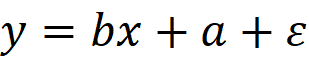In this guide, we’re going to show you how to do linear regression in Excel.

## Linear regression

Linear regression is a statistical analysis tool t find the correlation between two factors. It is calculated using the following formula, which shows how y is related to x:where,

• y: Dependent variable
• b: the slope of the regression line
• x: Independent variable
• a: y-intercept, a point where the regression line intersects y-axis
• ε: Error term, all other factors which influence the dependent variable (y) other than the independent variable (x).

Fortunately, you don't have to memorize this formula to apply it to your data model. Excel has a built-in formula for this.

## Doing linear regression in Excel

The easiest way to do linear regression in Excel is using a chart and a trendline. Let's take a look at how this works.

1. Select a cell in your data.
2. Create a scatter chart by following Insert > Insert Scatter Chart (X, Y) or Bubble Chart > Scatter.3. To add a trendline, click the big plus (+) button (Chart Elements) and select Linear under the Trendline item.4. Once the trendline is added, double-click on it to display the properties pane on the right side.
5. Make sure to enable Display equation on the chart.
6. (Optional) Open the Fill & Line section to modify your trendline. You can change its color or the line type.We changed the color of the trendline and adjusted the x-axis for better visibility.## What does the trendline tell us?

You can see how the two factors are related by the trendline slope.

• Positive slope (upward trend): The independent variable increases, the dependent variable also increases.
• Negative slope (downward trend): The independent variable increases, the dependent variable decreases.

Horizontal line: A horizontal line means that there is no correlation between the independent and dependent variables.